Search by Topic

Resources tagged with Factors and multiples similar to Rolling That Cube:

Filter by: Content type:
Age range:
Challenge level:

There are 112 results

Broad Topics > Numbers and the Number System > Factors and multiplesSweets in a Box

Age 7 to 11 Challenge Level:

How many different shaped boxes can you design for 36 sweets in one layer? Can you arrange the sweets so that no sweets of the same colour are next to each other in any direction?Mystery Matrix

Age 7 to 11 Challenge Level:

Can you fill in this table square? The numbers 2 -12 were used to generate it with just one number used twice.The Moons of Vuvv

Age 7 to 11 Challenge Level:

The planet of Vuvv has seven moons. Can you work out how long it is between each super-eclipse?It Figures

Age 7 to 11 Challenge Level:

Suppose we allow ourselves to use three numbers less than 10 and multiply them together. How many different products can you find? How do you know you've got them all?Multiply Multiples 1

Age 7 to 11 Challenge Level:

Can you complete this calculation by filling in the missing numbers? In how many different ways can you do it?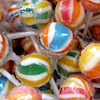Lots of Lollies

Age 5 to 7 Challenge Level:

Frances and Rishi were given a bag of lollies. They shared them out evenly and had one left over. How many lollies could there have been in the bag?Same Length Trains

Age 5 to 7 Challenge Level:

How many trains can you make which are the same length as Matt's, using rods that are identical?A Mixed-up Clock

Age 7 to 11 Challenge Level:

There is a clock-face where the numbers have become all mixed up. Can you find out where all the numbers have got to from these ten statements?Seven Flipped

Age 7 to 11 Challenge Level:

Investigate the smallest number of moves it takes to turn these mats upside-down if you can only turn exactly three at a time.Two Primes Make One Square

Age 7 to 11 Challenge Level:

Can you make square numbers by adding two prime numbers together?Multiplication Squares

Age 7 to 11 Challenge Level:

Can you work out the arrangement of the digits in the square so that the given products are correct? The numbers 1 - 9 may be used once and once only.Multiply Multiples 2

Age 7 to 11 Challenge Level:

Can you work out some different ways to balance this equation?One of Thirty-six

Age 5 to 7 Challenge Level:

Can you find the chosen number from the grid using the clues?Fractions in a Box

Age 7 to 11 Challenge Level:

The discs for this game are kept in a flat square box with a square hole for each. Use the information to find out how many discs of each colour there are in the box.Multiply Multiples 3

Age 7 to 11 Challenge Level:

Have a go at balancing this equation. Can you find different ways of doing it?Growing Garlic

Age 5 to 7 Challenge Level:

Ben and his mum are planting garlic. Use the interactivity to help you find out how many cloves of garlic they might have had.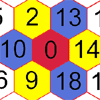Nineteen Hexagons

Age 5 to 7 Challenge Level:

In this maze of hexagons, you start in the centre at 0. The next hexagon must be a multiple of 2 and the next a multiple of 5. What are the possible paths you could take?Curious Number

Age 7 to 11 Challenge Level:

Can you order the digits from 1-3 to make a number which is divisible by 3 so when the last digit is removed it becomes a 2-figure number divisible by 2, and so on?Multiples Grid

Age 7 to 11 Challenge Level:

What do the numbers shaded in blue on this hundred square have in common? What do you notice about the pink numbers? How about the shaded numbers in the other squares?Neighbours

Age 7 to 11 Challenge Level:

In a square in which the houses are evenly spaced, numbers 3 and 10 are opposite each other. What is the smallest and what is the largest possible number of houses in the square?A Square Deal

Age 7 to 11 Challenge Level:

Complete the magic square using the numbers 1 to 25 once each. Each row, column and diagonal adds up to 65.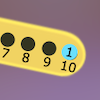Are You Well Balanced?

Age 5 to 7 Challenge Level:

Can you work out how to balance this equaliser? You can put more than one weight on a hook.Factor Lines

Age 7 to 14 Challenge Level:

Arrange the four number cards on the grid, according to the rules, to make a diagonal, vertical or horizontal line.Fitted

Age 7 to 11 Challenge Level:

Nine squares with side lengths 1, 4, 7, 8, 9, 10, 14, 15, and 18 cm can be fitted together to form a rectangle. What are the dimensions of the rectangle?Skip Counting

Age 5 to 7 Challenge Level:

Find the squares that Froggie skips onto to get to the pumpkin patch. She starts on 3 and finishes on 30, but she lands only on a square that has a number 3 more than the square she skips from.Tables Teaser

Age 5 to 7 Challenge Level:

How will you work out which numbers have been used to create this multiplication square?Grouping Goodies

Age 5 to 7 Challenge Level:

Pat counts her sweets in different groups and both times she has some left over. How many sweets could she have had?Scoring with Dice

Age 7 to 11 Challenge Level:

I throw three dice and get 5, 3 and 2. Add the scores on the three dice. What do you get? Now multiply the scores. What do you notice?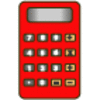Constant Counting

Age 5 to 7 Challenge Level:

You can make a calculator count for you by any number you choose. You can count by ones to reach 24. You can count by twos to reach 24. What else can you count by to reach 24?Biscuit Decorations

Age 5 to 7 Challenge Level:

Andrew decorated 20 biscuits to take to a party. He lined them up and put icing on every second biscuit and different decorations on other biscuits. How many biscuits weren't decorated?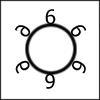Six in a Circle

Age 5 to 7 Challenge Level:

If there is a ring of six chairs and thirty children must either sit on a chair or stand behind one, how many children will be behind each chair?The Set of Numbers

Age 5 to 7 Challenge Level:

Can you place the numbers from 1 to 10 in the grid?Ip Dip

Age 5 to 11 Challenge Level:

"Ip dip sky blue! Who's 'it'? It's you!" Where would you position yourself so that you are 'it' if there are two players? Three players ...?Round and Round the Circle

Age 7 to 11 Challenge Level:

What happens if you join every second point on this circle? How about every third point? Try with different steps and see if you can predict what will happen.Tiling

Age 7 to 11 Challenge Level:

An investigation that gives you the opportunity to make and justify predictions.Gran, How Old Are You?

Age 7 to 11 Challenge Level:

When Charlie asked his grandmother how old she is, he didn't get a straightforward reply! Can you work out how old she is?Number Detective

Age 5 to 11 Challenge Level:

Follow the clues to find the mystery number.Little Squares

Age 5 to 7 Challenge Level:

Look at the squares in this problem. What does the next square look like? I draw a square with 81 little squares inside it. How long and how wide is my square?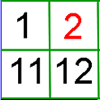What's Left?

Age 5 to 7 Challenge Level:

Use this grid to shade the numbers in the way described. Which numbers do you have left? Do you know what they are called?Times Tables Shifts

Age 7 to 11 Challenge Level:

In this activity, the computer chooses a times table and shifts it. Can you work out the table and the shift each time?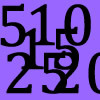Doubling Fives

Age 5 to 7 Challenge Level:

This activity focuses on doubling multiples of five.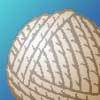Mrs Trimmer's String

Age 5 to 7 Challenge Level:

Can you help the children in Mrs Trimmer's class make different shapes out of a loop of string?Pebbles

Age 7 to 11 Challenge Level:

Place four pebbles on the sand in the form of a square. Keep adding as few pebbles as necessary to double the area. How many extra pebbles are added each time?Making Pathways

Age 7 to 11 Challenge Level:

Can you find different ways of creating paths using these paving slabs?Sets of Four Numbers

Age 7 to 11 Challenge Level:

There are ten children in Becky's group. Can you find a set of numbers for each of them? Are there any other sets?Sorting Numbers

Age 5 to 7 Challenge Level:

Can you sort numbers into sets? Can you give each set a name?Domino Pick

Age 5 to 7 Challenge Level:

Are these domino games fair? Can you explain why or why not?Abundant Numbers

Age 7 to 11 Challenge Level:

48 is called an abundant number because it is less than the sum of its factors (without itself). Can you find some more abundant numbers?Being Resilient - Primary Number

Age 5 to 11 Challenge Level:

Number problems at primary level that may require resilience.Being Collaborative - Primary Number

Age 5 to 11 Challenge Level:

Number problems at primary level to work on with others.